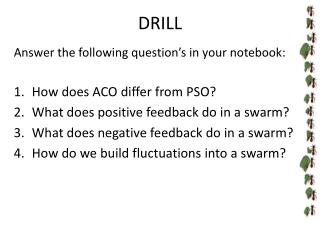DownloadDownload PresentationDRILL

# DRILL

Download Presentation## DRILL

- - - - - - - - - - - - - - - - - - - - - - - - - - - E N D - - - - - - - - - - - - - - - - - - - - - - - - - - -
##### Presentation Transcript

1. DRILL Answer the following question’s in your notebook: • How does ACO differ from PSO? • What does positive feedback do in a swarm? • What does negative feedback do in a swarm? • How do we build fluctuations into a swarm?

2. Swarm Theory: “The Wisdom of Crowds” Creating a Swarm (pt 2)

3. Models for Swarming Behavior Ant Colony Optimization (ACO) Particle Swarm Optimization (PSO) Birds are excellent at finding multiplesources of food simultaneously Birds broadcast information locally • Ants are great at finding the closest source of food • Ants use pheromones to communicate information • Works best for finding continuous solutions • Works best for finding discrete solutions

4. Swarm Theory: Setting it up To solve a problem using swarming techniques you will have to: • Define the domain • Create an algorithm • Write instructions for the agent • Define the criteria for completion

5. Swarm Theory: Probability Computers don’t “think” the same way we do. How do we tell a computer to send an agent towards the most likely solution? We use an understanding of probability and randomly generated numbers to accomplish the task.

6. Swarm Theory: Probability Experiment – an act for which the outcome is uncertain (e.g. coin toss, dice rolls, survey) Probability – the likelihood that something will occur or be true Sample Space – the set of all possible outcomes for an experiment Event – any subset of the sample space

7. Swarm Theory: Probability Probability – the likelihood that something will occur or be true The sum of all probabilities for all events in the sample space must sum to 1.

8. Swarm Theory: Probability If a box contains 4 red marbles and 6 blue marbles, what are the odds of picking a red marble from the box without looking? Experiment: Picking a marble Sample Space: R R R R B B B B B B Event, E: Pick any red marble

9. Swarm Theory: Probability Random Number – a number drawn from a set of numbers where each number is equally likely to be drawn Random Number Generators – a deterministic algorithm that generates a string of random numbers (better called pseudo-random)

10. Swarm Theory: Probability A random number between 0 and 1 is drawn in order to be placed into a bin. If the number is between 0 and 0.5, it will be placed into Bin#1. If it is greater than 0.5 and less than 1, it will be placed into Bin#2. What are the odds that it will be placed into Bin#2?

11. Defining the Domain Domain – the set of all possible input values to a function. In this case, the domain is spatial: 8x8 grid of spaces

12. Creating an Algorithm Algorithm – a step by step procedure for solving a problem, usually iterative • Positive Feedback –> • Negative Feedback –> • Fluctuation –> • Multiple Interactions –> “Fastest Way to Food” • Scent trail • Smell fades • Random #’s • Determines direction • Scent increases chance of direction being chosen • Iteration

13. Building the Agent Each agent in a swarm is given a set of simple instructions that require it to search the domain, test its surroundings and communicate results. • Explore grid and look for food • Leave scent trail as you return to nest • Share information with nest “Fastest Way to Food”

14. Setting the Threshold Every swarm intelligence program must know when to stop…that stopping point is called the threshold. • End if the path to food remains constant • End if the path to food continues to change after more than N iterations *N is a number that you choose and can play with. “Fastest Way to Food”

15. Swarm Theory • Homework: • Read over tomorrow’s lecture (U1 L5.ppt) • Pop quiz is possible • Set up a swarm intelligence to solve this problem: • The CEO of a large company calls a meeting of her executives to decide where to relocate their offices. There are five spaces in a large building nearby. All of the spaces could serve as a new location, but one of them is optimal. How should the executives choose their new office space (use swarm intelligence)?